# Simple Harmonic Motion

## Simple Harmonic Motion of Class 11

### Simple Harmonic Motion

Let us consider an oscillatory particle along a straight line whose potential energy function varies as

U(x) = 1/2kx2     (13.3)

where k is a constant

The force acting on the particle is given by

F =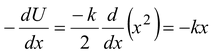(13.4)

Such an oscillatory motion in which restoring force acting on the particle is directly proportional to the displacement from the equilibrium position is called Simple Harmonic Motion.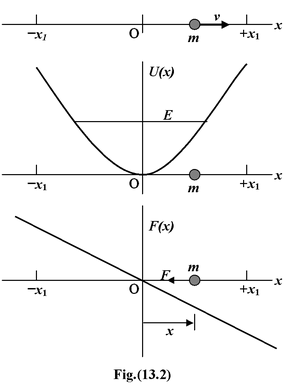The potential energy function of such a particle is represented by a symmetric curve as shown in the figure(13.2).

Note that the limits of oscillation are equally spaced about the equilibrium position. This is not true for the motion shown in figure(13.1), which is harmonic, but not simple harmonic.

Applying Newton’s Second Law in equation (13.4), we get

F = ma = -kx

For the acceleration we can put

a =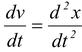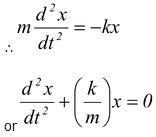(13.5)

The equation(13.5) is called the differential equation of SHM.

 It gives a relation between a function of the time x(t) and its second derivative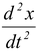. To find the position of the particle as a function of the time, we must find a function x(t) which satisfies this relation. It is obvious from a simple experiment as depicted in fig.(13.3), in which an oscillating particle traces a sinusoidal curve on a moving strip of paper. In general, the equation of a simple harmonic motion may be represented by any of the following function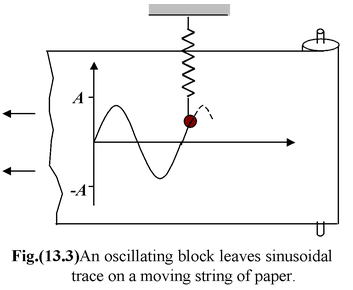x = A sin (ω t + φ)(13.6 a)

x = A cos (ω t + φ) (13.6 b)

x = A sin ωt + B cos ωt (13.6 c)

Although all the above three equations are the solution of the differential equation (13.5) but we will be using x = A sin (ωt + φ) as the general equation of SHM.

### Characteristics of SHM

−The amplitude A is constant

−The frequency and period are independent of the amplitude.

This implies large oscillations have the same period as small ones.

 In equation (13.6 a), the argument (ωt + φ) is called the phase, where φ is called the phase constant. Both the phase and the phase constant are measured in radians. The value of φ depends on the position wherefrom we start measuring time.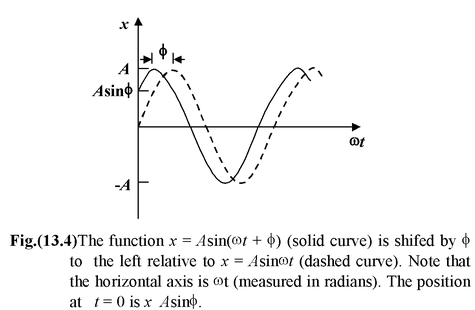The characteristic of the differential equation (13.5) is that it does not represent one single motion but a group or family of possible motions which have some features in common but differ in other ways. In this case ω is common to all the allowed motions, but A and φ may differ among them. The amplitude A and the phase constant φ of the oscillation are determined by the initial position and speed of the particle. These two initial conditions will specify A and φ exactly.

 One very important distinctive feature of SHM is the relation between the displacement, velocity and acceleration of oscillatory particle. x = A sin ωt (13.7) v = dx/dt  = ωA cos ωt = ωA sin (ωt + π/2) (13.8) a ==  -ω2A sin ωt = ω2A sin (ωt + π) (13.9) Substituting the value of x in the above equation or= −ω2x   or+ ω2x = 0 (13.10) This is the standard differential equation of SHM. Notice that the maximum displacement is A, the maximum speed is ωA, and the maximum acceleration is ω2A. The displacement, velocity and acceleration versus time graphs have been plotted in figure(13.5).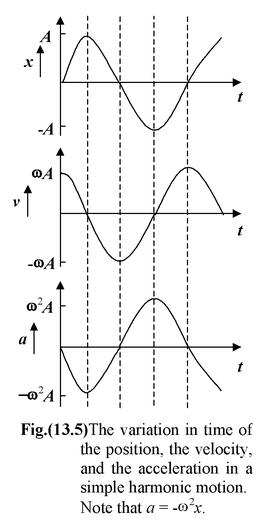The velocity function is π/2 ahead of the displacement function and the acceleration function is π/2 ahead of the velocity function.

## Conditions of Simple Harmonic Motion

For SHM to occur, three conditions must be satisfied.

1.There must be a position of stable equilibrium

At the stable equilibrium potential energy is minimum.

That is,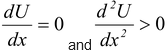(13.11)

2.There must be no dissipation of energy

3.The acceleration is proportional to the displacement and opposite in direction.

That is, a = −ω2x(13.12)

Example: 13.1

The position of a particle moving along x – axis is given by

x = 0.08 sin (12t + 0.3) m

where t is in second.

(a)What are the amplitude and period of the motion?

(b)Determine the position, velocity, and acceleration at t = 0.6 s.

Solution

(a)On comparing the given equation with the form in equation (13.6 a), we see that the amplitude is A = 0.08 m and the angular frequency ω = 12 rad/s. Thus, the period is
T = 2π/ω = 0.523 s.

(b)The velocity and acceleration at any time are given by

v = dx/dt  = 0.96 cos (12 t + 0.3) m/s

a = dv/dt =  −11.52 sin (12 t + 0.3) m/s2

At t = 0.6 s, the phase of motion is (12 × 0.6 + 0.3) = 7.5 rad.

When this is used in the above expressions, we find

x = 0.075 m, v = 0.333 m/s, and a = -10.8 m/s2.

 13.2Simple Harmonic Motion Let us consider an oscillatory particle along a straight line whose potential energy function varies as U(x) = 1/2kx2   (13.3) where k is a constant The force acting on the particle is given by F =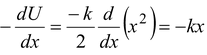(13.4) Such an oscillatory motion in which restoring force acting on the particle is directly proportional to the displacement from the equilibrium position is called Simple Harmonic Motion.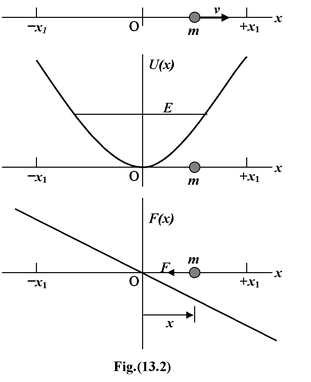The potential energy function of such a particle is represented by a symmetric curve as shown in the figure(13.2).

Note that the limits of oscillation are equally spaced about the equilibrium position. This is not true for the motion shown in figure(13.1), which is harmonic, but not simple harmonic.

Applying Newton’s Second Law in equation (13.4), we get

F = ma = -kx

For the acceleration we can put

a =(13.5)

The equation(13.5) is called the differential equation of SHM.

 It gives a relation between a function of the time x(t) and its second derivative. To find the position of the particle as a function of the time, we must find a function x(t) which satisfies this relation. It is obvious from a simple experiment as depicted in fig.(13.3), in which an oscillating particle traces a sinusoidal curve on a moving strip of paper. In general, the equation of a simple harmonic motion may be represented by any of the following functionx = A sin (ω t + φ)(13.6 a)

x = A cos (ω t + φ) (13.6 b)

x = A sin ωt + B cos ωt (13.6 c)

Although all the above three equations are the solution of the differential equation (13.5) but we will be using x = A sin (ωt + φ) as the general equation of SHM.

Characteristics of SHM

−The amplitude A is constant

−The frequency and period are independent of the amplitude.

This implies large oscillations have the same period as small ones.

 In equation (13.6 a), the argument (ωt + φ) is called the phase, where φ is called the phase constant. Both the phase and the phase constant are measured in radians. The value of φ depends on the position wherefrom we start measuring time.The characteristic of the differential equation (13.5) is that it does not represent one single motion but a group or family of possible motions which have some features in common but differ in other ways. In this case ω is common to all the allowed motions, but A and φ may differ among them. The amplitude A and the phase constant φ of the oscillation are determined by the initial position and speed of the particle. These two initial conditions will specify A and φ exactly.

 One very important distinctive feature of SHM is the relation between the displacement, velocity and acceleration of oscillatory particle. x = A sin ωt (13.7) v = dx/dt  = ωA cos ωt = ωA sin (ωt + π/2) (13.8) a ==  -ω2A sin ωt = ω2A sin (ωt + π) (13.9) Substituting the value of x in the above equation or= −ω2x   or+ ω2x = 0 (13.10) This is the standard differential equation of SHM. Notice that the maximum displacement is A, the maximum speed is ωA, and the maximum acceleration is ω2A. The displacement, velocity and acceleration versus time graphs have been plotted in figure(13.5).The velocity function is π/2 ahead of the displacement function and the acceleration function is π/2 ahead of the velocity function.

Conditions of Simple Harmonic Motion

For SHM to occur, three conditions must be satisfied.

1.There must be a position of stable equilibrium

At the stable equilibrium potential energy is minimum.

That is,(13.11)

2.There must be no dissipation of energy

3.The acceleration is proportional to the displacement and opposite in direction.

That is, a = −ω2x(13.12)

Example: 13.1

The position of a particle moving along x – axis is given by

x = 0.08 sin (12t + 0.3) m

where t is in second.

(a)What are the amplitude and period of the motion?

(b)Determine the position, velocity, and acceleration at t = 0.6 s.

Solution

(a)On comparing the given equation with the form in equation (13.6 a), we see that the amplitude is A = 0.08 m and the angular frequency ω = 12 rad/s. Thus, the period is
T = 2π/ω = 0.523 s.

(b)The velocity and acceleration at any time are given by

v = dx/dt  = 0.96 cos (12 t + 0.3) m/s

a = dv/dt =  −11.52 sin (12 t + 0.3) m/s2

At t = 0.6 s, the phase of motion is (12 × 0.6 + 0.3) = 7.5 rad.

When this is used in the above expressions, we find

x = 0.075 m, v = 0.333 m/s, and a = -10.8 m/s2.